Next: Surface Harmonics and Solid Up: Terrestrial Ocean Tides Previous: Decomposition of Tide Generating

# Expansion of Tide Generating Potential

Suppose that, in the,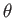,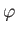frame, the moon's orbit is a Keplerian ellipse of major radius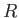, and eccentricity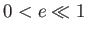, lying in a fixed plane that is inclined at an angle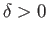to the plane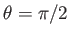. Thus, the closest and furthest distance between the centers of the moon and the planet are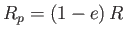and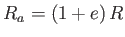, respectively. It follows that (Fitzpatrick 2013)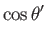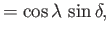(12.29)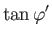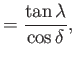(12.30)

where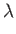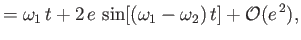(12.31)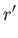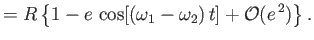(12.32)

Here, it is assumed that the closest point on the moon's orbit to the center of the planet corresponds to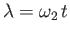, where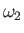is the uniform precession rate of this point. [It is necessary to include such precession in our analysis because the Moon's perigee precesses steadily in such a manner that it completes an orbit about the Earth once every 8.85 years. This effect is caused by the perturbing influence of the Sun (Fitzpatrick 2013).] Suppose that the inclination of the moon's orbit to the planet's equatorial plane,, is relatively small, so that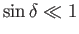. It follows that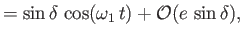(12.33)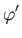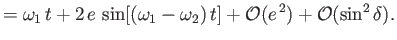(12.34)

Thus, Equations (12.22)-(12.24), (12.28), and (12.32)-(12.34) can be combined to give the following expression for the tide generating potential due to a moon in a low-eccentricity, low-inclination orbit: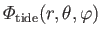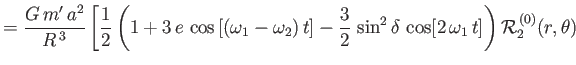(12.35)

Here, we have retained a term proportional to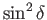in the previous expression, despite the fact that we are formally neglecting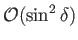terms, because the term in question gives rise to important fortnightly tides on the Earth.Next: Surface Harmonics and Solid Up: Terrestrial Ocean Tides Previous: Decomposition of Tide Generating
Richard Fitzpatrick 2016-03-31